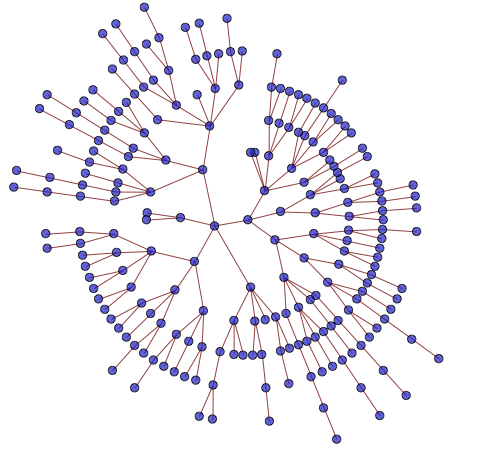# Combinatorial Algorithms (RM35KOA)

## Course overviewThis course focuses on the problems and algorithms of combinatorial optimization (often called discrete optimization). We emphasize the practical use of optimization techniques and algorithms based on graphs, integer linear programming, heuristics, approximation algorithms, and state-space search methods. You will learn how to formulate the problem using existing formalisms, and you will obtain experience with the existing algorithms solving them. We demonstrate the use of combinatorial optimization algorithms on a large variety of real-life applications, e.g., logistics, transportation, planning of human resources, scheduling in production lines, message routing, and scheduling in parallel computers.

## Lectures

Week No. Dates Title Lecturer Handouts Notes
1 14.2.-20.2. Introduction of Basic Terms, Example Applications ZH https://rtime.felk.cvut.cz/~hanzalek/KO/Basic_e.pdf Intro
2 21.2.-27.2. Computational Complexity AN https://rtime.ciirc.cvut.cz/~novakan9/KOA/Complexity_e.pdf
3 28.2.-6.3. Integer Linear Programming - Algorithms ZH https://rtime.felk.cvut.cz/~hanzalek/KO/ILP_e.pdf ILP-I
4 7.3.-13.3. Integer Linear Programming - Problem Formulations ZH https://rtime.felk.cvut.cz/~hanzalek/KO/ILP_e.pdf ILP-II
5 14.3.-20.3. Shortest Paths ZH https://rtime.felk.cvut.cz/~hanzalek/KO/SPT_e.pdf SP-I
SP-II
SP-III
6 21.3.-27.3. Problem Formulation by Shortest Paths ZH https://rtime.felk.cvut.cz/~hanzalek/KO/SPT_e.pdf Test 1.
7 28.3.-3.4. Flows and Cuts - Algorithms and Problem Formulation ZH https://rtime.felk.cvut.cz/~hanzalek/KO/Flows_e.pdf Flows-I
Flows-II
Flows-III
8 4.4.-10.4. Bipartite Matching. Multi-commodity Network Flows ZH https://rtime.felk.cvut.cz/~hanzalek/KO/Flows_e.pdf
9 11.4.-17.4. Knapsack Problem, Pseudo-polynomial and Approximation Algorithms ZH https://rtime.felk.cvut.cz/~hanzalek/KO/knapsack_e.pdf Knapsack-I
10 18.4.-24.4. Public holiday
11 25.4.-1.5. Traveling Salesman Problem and Approximation Algorithms ZH https://rtime.felk.cvut.cz/~hanzalek/KO/TSP_e.pdf Test 2.
TSP-I
12 2.5.-8.5. Mono-processor Scheduling ZH https://rtime.felk.cvut.cz/~hanzalek/KO/sched_e.pdf Sched-I
Sched-II
Sched-III
Sched--IV
13 9.5.-15.5 Scheduling on Parallel Processors ZH https://rtime.felk.cvut.cz/~hanzalek/KO/sched_e.pdf
14 16.5.-22.5. Constraint Programming ZH https://rtime.felk.cvut.cz/~hanzalek/KO/cp_e.pdf CP-I

Materials by topic

Title Handouts Stream Introduction https://rtime.felk.cvut.cz/~hanzalek/KO/Basic_e.pdf Intro Complexity https://rtime.ciirc.cvut.cz/~novakan9/KOA/Complexity_e.pdf Integer Linear Programming https://rtime.felk.cvut.cz/~hanzalek/KO/ILP_e.pdf ILP-I ILP-II Shortest paths https://rtime.felk.cvut.cz/~hanzalek/KO/SPT_e.pdf SP-I SP-II SP-III Network flows https://rtime.felk.cvut.cz/~hanzalek/KO/Flows_e.pdf Flows-I Flows-II Flows-III Knapsack https://rtime.felk.cvut.cz/~hanzalek/KO/knapsack_e.pdf Knapsack-I Traveling Salesman Problem https://rtime.felk.cvut.cz/~hanzalek/KO/TSP_e.pdf TSP-I Scheduling https://rtime.felk.cvut.cz/~hanzalek/KO/sched_e.pdf Sched-I Sched-II Sched-III Sched--IV Constraint Programming https://rtime.felk.cvut.cz/~hanzalek/KO/cp_e.pdf CP-I

Students may receive a total of 100 points. The final exam is worth 50% of the grade, while the other 50% can be obtained during the semester.

The points are distributed according to the following table.

 Category Maximum points Semester Homeworks (3 assignments) 15 Theoretical tests (two tests, 10 points each) 20 Semester project 15 Minimum points Total semester 1) 50 30 Final exam 2) Written part 40 20 Oral exam 10 0 Total 100 50

1) To get an ungraded assessment the following requirements have to be met:

• successfully solve all homework assignments (i.e., accepted by BRUTE evaluation as correct)
• obtain at least 30 from 50 points

The last date of awarding the ungraded assessments is 22. 5. 2022. If you fail to fulfill the requirements by then, you will also fail the entire class.

2) Only the students, who obtained the ungraded assessment are allowed to take the exam. The exam constitutes of the written part (40 points) and oral exam (10 points). To pass the written exam, it is necessary to get at least 20 points. The oral exam is mandatory.

Points [0,50) [50,60) [60,70) [70,80) [80,90) [90,100]
Grade F E D C B A

### Assessment recognition

The following applies only to students who got an ungraded assessment in the previous year (all other students have to undergo the course from scratch).

Although the assessments are not fully recognized, the students may opt-in to transfer some points from the previous year to the current one (they may also undergo the course from scratch to get a higher number of points). The following rules apply

1. Theoretical tests, practical test and the homeworks have to be repeated.
2. Students may transfer points from the semester project. If the grading table of the course changes between the years, the points are scaled accordingly. It is also possible to work on the same topic to increase/decrease the number of received points.
3. The minimum number of points required to get an ungraded assessment is always taken from the current year grading table.